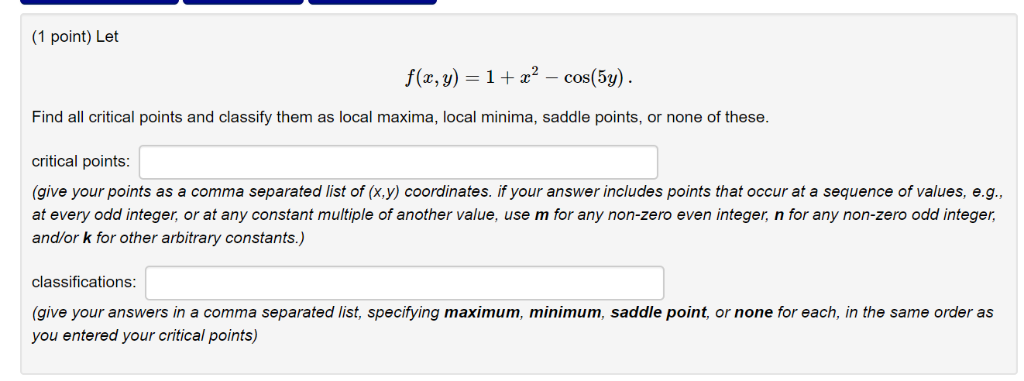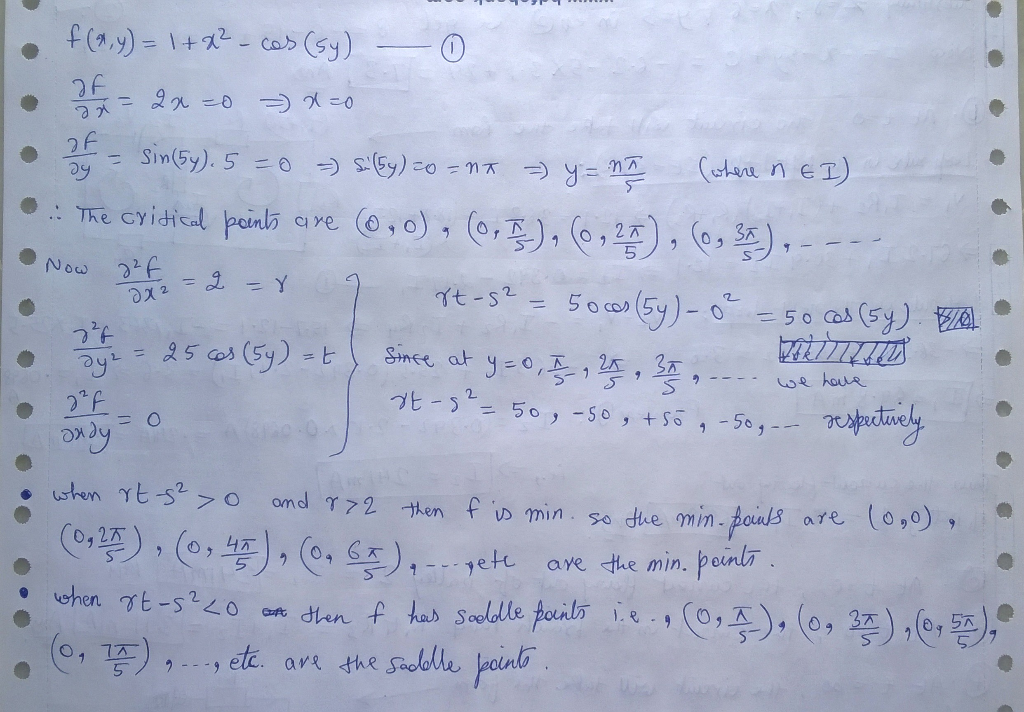# Let f(x,y)=1+x2−cos(5y). Find all critical points and classify them as local maxima, local minima, saddle points, or non...

Let f(x,y)=1+x2−cos(5y). Find all critical points and classify them as local maxima, local minima, saddle points, or none of these.(1 point) Let Find all critical points and classify them as local maxima, local minima, saddle points, or none of these. critical points (give your points as a comma separated list of (x,y) coordinates. if your answer includes points that occur at a sequence of values, e.g., at every odd integer, or at any constant multiple of another value, use m for any non-zero even integer, n for any non-zero odd integer, and/or k for other arbitrary constants.) classifications (give your answers in a comma separated list, specifying maximum, minimum, saddle point, or none for each, in the same order as you entered your critical points)##### Add Answer of: Let f(x,y)=1+x2−cos(5y). Find all critical points and classify them as local maxima, local minima, saddle points, or non...
More Homework Help Questions Additional questions in this topic.